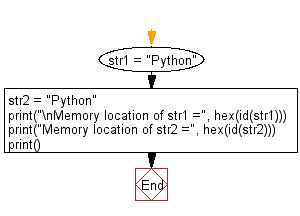﻿ Python: Prove that two string variables of same value point same memory location - w3resource# Python: Prove that two string variables of same value point same memory location

## Python Basic: Exercise-117 with Solution

Write a Python program to prove that two string variables of same value point same memory location.

Sample Solution:-

Python Code:

``````str1 = "Python"
str2 = "Python"

print("\nMemory location of str1 =", hex(id(str1)))
print("Memory location of str2 =", hex(id(str2)))
print()
```
```

Sample Output:

```Memory location of str1 = 0x7f8af3e89f10
Memory location of str2 = 0x7f8af3e89f10
```

Flowchart:## Visualize Python code execution:

The following tool visualize what the computer is doing step-by-step as it executes the said program:

Python Code Editor:

Have another way to solve this solution? Contribute your code (and comments) through Disqus.

What is the difficulty level of this exercise?

Test your Python skills with w3resource's quiz

﻿

## Python: Tips of the Day

List comprehension:

```>>> m = [x ** 2 for x in range(5)]
>>> m
[0, 1, 4, 9, 16]
```